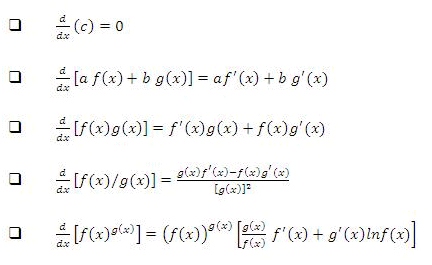Courses

Revision Notes - Differentiability Notes | Study JEE Revision Notes - JEE

JEE: Revision Notes - Differentiability Notes | Study JEE Revision Notes - JEE

The document Revision Notes - Differentiability Notes | Study JEE Revision Notes - JEE is a part of the JEE Course JEE Revision Notes.
All you need of JEE at this link: JEE
• The derivative of f, denoted by f'(x) is given by f'(x) = lim?x→0 (? y)/(? x) = dy/dx
• The right hand derivative of f at x = a is denoted by f'(a+) and is given by f'(a+) = limh→0+(f(a+h)-f(a))/h
• The left hand derivative of f at x = a is denoted by f'(a-) and is given by f'(a-) = limh→0-  (f(a-h)-f(a))/-h
• For a function to be differentiable at x=a, we should have f'(a-)=f'(a+) i.e. limh→0 (f (a-h)-f (a))/ (-h) = limh→0 (f (a+h)-f (a))/h.
• limh→0 sin 1/h fluctuates between -1 and 1.
• If at a particular point say x =a, we have f'(a+) = t1 (a finite number) and f'(a-) = t2 (a finite number) and if t1 ≠ t2, then f' (a) does not exist, but f(x) is a continuous function at x = a.
• Continuity and differentiability are quite interrelated.
Differentiability alwaysimpliescontinuity but the converse is not true. This means that a differentiable function is always continuous but if a function is continuous it may or may not be differentiable.
Some basic formulae:• If a function is not derivable at a point, it need not imply that it is discontinuous at that point. But, however, discontinuity at a point necessarily implies non-derivability.
• In case, a function is not differentiable but is continuous at a particular point say x = a, then it geometrically implies a sharp corner at x = a.
• A function f is said to be derivable over a closed interval [a, b] if :
1. For the points a and b, f'(a+) and f'(b-) exist and
2. For ant point c such that a < c < b, f'(c+)and f'(c-) exist and are equal.
• If y = f(u) and u = g(x), then dy/dx = dy/du.du/dx = f'(g(x)) g'(x). This method is also termed as the chain rule.
• For composite functions, differentiation is carried out in this way:
If y = [f(x)]n, then we put u = f(x). So that y = un. Then by chain rule:
dy/dx = dy/du.du/dx = nu(n-1)f' (x) = [f(x)](n-1) f' (x)
• Differential calculus problems involving parametric functions:
If x and y are functions of parameter t, first find dx/dt and dy/dt separately. Then dy/dx=(dy/dt)/(dx/dt).
• If the functions f(x) and g(x) are derivable at x = a, then the following functions are also derivable:
1.f(x) + g(x)
2.f(x) - g(x)
3.f(x) . g(x)
4.f(x) / g(x), provided g(a) ≠ 0
• If the function f(x) is differentiable at x =a while g(x) is not derivable at x = a, then the product function f(x). g(x) can still be differentiable at x = a.
• Even if both the functions f(x) and g(x) are not differentiable at x = a, the product function f(x).g(x) can still be differentiable at x = a.
• Even if both the functions f(x) and g(x) are not derivable at x = a, the sum function f(x) + g(x) can still be differentiable at x = a.
If function f(x) is derivable at x = a, this need not imply that f'(x) is continuous at x = a.
The document Revision Notes - Differentiability Notes | Study JEE Revision Notes - JEE is a part of the JEE Course JEE Revision Notes.
All you need of JEE at this link: JEEUse Code STAYHOME200 and get INR 200 additional OFF Use Coupon Code

Top Courses for JEEJEE Revision Notes

170 docs

Top Courses for JEETrack your progress, build streaks, highlight & save important lessons and more!

,

,

,

,

,

,

,

,

,

,

,

,

,

,

,

,

,

,

,

,

,

;